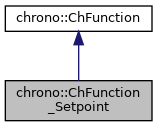chrono::ChFunction_Setpoint Class Reference

## Description

Function that returns Y from an externally-provided value, as a ZOH (zero order hold) block.

This means that the Y value does NOT change if you call Get_y(double x) with different values of x, unless you keep the setpoint Y updated via multiple calls to SetSetpoint(), for example calling SetSetpoint() at each timestep in the simulation loop. Also first two derivatives (speed, accel.) will persist until next SetSetpoint() call. Function of this class are most often functions of time.

#include <ChFunction_Setpoint.h>

Inheritance diagram for chrono::ChFunction_Setpoint:[legend]
Collaboration diagram for chrono::ChFunction_Setpoint:[legend]

## Public Member Functions

ChFunction_Setpoint (const ChFunction_Setpoint &other)

virtual ChFunction_SetpointClone () const override
"Virtual" copy constructor (covariant return type).

virtual double Get_y (double x) const override
Return the y value of the function, at position x.

virtual double Get_y_dx (double x) const override
Return the dy/dx derivative of the function, at position x. More...

virtual double Get_y_dxdx (double x) const override
Return the ddy/dxdx double derivative of the function, at position x. More...

virtual void SetSetpoint (double setpoint, double x)
Set the setpoint, and compute its derivatives (speed, acceleration) automatically by backward differentiation (only if x is called at increasing small steps). More...

virtual void SetSetpointAndDerivatives (double setpoint, double setpoint_dx, double setpoint_dxdx)
Set the setpoint, and also its derivatives. More...

double GetSetpoint ()
Get the last set setpoint.

virtual void Update (const double x) override
Update could be implemented by children classes, ex. to launch callbacks.

virtual void ArchiveOUT (ChArchiveOut &marchive) override
Method to allow serialization of transient data to archives.

virtual void ArchiveIN (ChArchiveIn &marchive) override
Method to allow de-serialization of transient data from archives.Public Member Functions inherited from chrono::ChFunction
ChFunction (const ChFunction &other)

virtual FunctionType Get_Type () const
Return the unique function type identifier.

virtual double Get_weight (double x) const
Return the weight of the function (useful for applications where you need to mix different weighted ChFunctions)

virtual void Estimate_x_range (double &xmin, double &xmax) const
Return an estimate of the range of the function argument. More...

virtual void Estimate_y_range (double xmin, double xmax, double &ymin, double &ymax, int derivate) const
Return an estimate of the range of the function value. More...

virtual double Get_y_dN (double x, int derivate) const
Return the function derivative of specified order at the given point. More...

virtual double Compute_max (double xmin, double xmax, double sampling_step, int derivate) const
Compute the maximum of y(x) in a range xmin-xmax, using a sampling method.

virtual double Compute_min (double xmin, double xmax, double sampling_step, int derivate) const
Compute the minimum of y(x) in a range xmin-xmax, using a sampling method.

virtual double Compute_mean (double xmin, double xmax, double sampling_step, int derivate) const
Compute the mean value of y(x) in a range xmin-xmax, using a sampling method.

virtual double Compute_sqrmean (double xmin, double xmax, double sampling_step, int derivate) const
Compute the square mean val. of y(x) in a range xmin-xmax, using sampling.

virtual double Compute_int (double xmin, double xmax, double sampling_step, int derivate) const
Compute the integral of y(x) in a range xmin-xmax, using a sampling method.

virtual double Get_Ca_pos () const
Computes the positive acceleration coefficient (inherited classes should customize this).

virtual double Get_Ca_neg () const
Compute the positive acceleration coefficient (inherited classes should customize this).

virtual double Get_Cv () const
Compute the speed coefficient (inherited classes must customize this).

virtual int HandleNumber () const
Return the number of handles of the function.

virtual bool HandleAccess (int handle_id, double mx, double my, bool set_mode)
Get the x and y position of handle, given identifier. More...

virtual int FilePostscriptPlot (ChFile_ps *m_file, int plotY, int plotDY, int plotDDY)
Plot function in graph space of the ChFile_ps postscript file where zoom factor, centering, color, thickness etc. More...

virtual int FileAsciiPairsSave (ChStreamOutAscii &m_file, double xmin=0, double xmax=1, int msamples=200)
Save function as X-Y pairs separated by space, with CR at each pair, into an ASCII file. More...

## Additional Inherited MembersPublic Types inherited from chrono::ChFunction
enum  FunctionType {
FUNCT_CUSTOM, FUNCT_CONST, FUNCT_CONSTACC, FUNCT_DERIVE,
FUNCT_FILLET3, FUNCT_INTEGRATE, FUNCT_MATLAB, FUNCT_MIRROR,
FUNCT_MOCAP, FUNCT_NOISE, FUNCT_OPERATION, FUNCT_OSCILLOSCOPE,
FUNCT_POLY, FUNCT_POLY345, FUNCT_RAMP, FUNCT_RECORDER,
FUNCT_REPEAT, FUNCT_SEQUENCE, FUNCT_SIGMA, FUNCT_SINE,
FUNCT_LAMBDA
}
Enumeration of function types.

## ◆ Get_y_dx()

 virtual double chrono::ChFunction_Setpoint::Get_y_dx ( double x ) const
inlineoverridevirtual

Return the dy/dx derivative of the function, at position x.

Note that inherited classes may also avoid overriding this method, because this base method already provide a general-purpose numerical differentiation to get dy/dx only from the Get_y() function. (however, if the analytical derivative is known, it may better to implement a custom method).

Reimplemented from chrono::ChFunction.

## ◆ Get_y_dxdx()

 virtual double chrono::ChFunction_Setpoint::Get_y_dxdx ( double x ) const
inlineoverridevirtual

Return the ddy/dxdx double derivative of the function, at position x.

Note that inherited classes may also avoid overriding this method, because this base method already provide a general-purpose numerical differentiation to get ddy/dxdx only from the Get_y() function. (however, if the analytical derivative is known, it may be better to implement a custom method).

Reimplemented from chrono::ChFunction.

## ◆ SetSetpoint()

 void chrono::ChFunction_Setpoint::SetSetpoint ( double setpoint, double x )
virtual

Set the setpoint, and compute its derivatives (speed, acceleration) automatically by backward differentiation (only if x is called at increasing small steps).

All values will persist indefinitely until next call.

## ◆ SetSetpointAndDerivatives()

 virtual void chrono::ChFunction_Setpoint::SetSetpointAndDerivatives ( double setpoint, double setpoint_dx, double setpoint_dxdx )
inlinevirtual

Set the setpoint, and also its derivatives.

All values will persist indefinitely until next call.

The documentation for this class was generated from the following files:
• /builds/uwsbel/chrono/src/chrono/motion_functions/ChFunction_Setpoint.h
• /builds/uwsbel/chrono/src/chrono/motion_functions/ChFunction_Setpoint.cpp